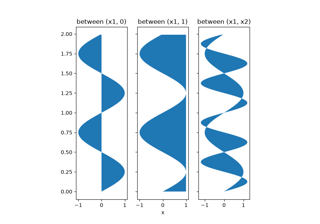# matplotlib.axes.Axes.fill_betweenx#

Axes.fill_betweenx(y, x1, x2=0, where=None, step=None, interpolate=False, *, data=None, **kwargs)[source]#

Fill the area between two vertical curves.

The curves are defined by the points (y, x1) and (y, x2). This creates one or multiple polygons describing the filled area.

You may exclude some vertical sections from filling using where.

By default, the edges connect the given points directly. Use step if the filling should be a step function, i.e. constant in between y.

Parameters:
yarray (length N)

The y coordinates of the nodes defining the curves.

x1array (length N) or scalar

The x coordinates of the nodes defining the first curve.

x2array (length N) or scalar, default: 0

The x coordinates of the nodes defining the second curve.

wherearray of bool (length N), optional

Define where to exclude some vertical regions from being filled. The filled regions are defined by the coordinates `y[where]`. More precisely, fill between `y[i]` and `y[i+1]` if `where[i] and where[i+1]`. Note that this definition implies that an isolated True value between two False values in where will not result in filling. Both sides of the True position remain unfilled due to the adjacent False values.

interpolatebool, default: False

This option is only relevant if where is used and the two curves are crossing each other.

Semantically, where is often used for x1 > x2 or similar. By default, the nodes of the polygon defining the filled region will only be placed at the positions in the y array. Such a polygon cannot describe the above semantics close to the intersection. The y-sections containing the intersection are simply clipped.

Setting interpolate to True will calculate the actual intersection point and extend the filled region up to this point.

step{'pre', 'post', 'mid'}, optional

Define step if the filling should be a step function, i.e. constant in between y. The value determines where the step will occur:

• 'pre': The y value is continued constantly to the left from every x position, i.e. the interval `(x[i-1], x[i]]` has the value `y[i]`.

• 'post': The y value is continued constantly to the right from every x position, i.e. the interval `[x[i], x[i+1])` has the value `y[i]`.

• 'mid': Steps occur half-way between the x positions.

Returns:
`PolyCollection`

A `PolyCollection` containing the plotted polygons.

Other Parameters:
dataindexable object, optional

If given, the following parameters also accept a string `s`, which is interpreted as `data[s]` (unless this raises an exception):

y, x1, x2, where

**kwargs

All other keyword arguments are passed on to `PolyCollection`. They control the `Polygon` properties:

Property

Description

`agg_filter`

a filter function, which takes a (m, n, 3) float array and a dpi value, and returns a (m, n, 3) array and two offsets from the bottom left corner of the image

`alpha`

array-like or scalar or None

`animated`

bool

`antialiased` or aa or antialiaseds

bool or list of bools

`array`

array-like or None

`capstyle`

`CapStyle` or {'butt', 'projecting', 'round'}

`clim`

(vmin: float, vmax: float)

`clip_box`

`Bbox`

`clip_on`

bool

`clip_path`

Patch or (Path, Transform) or None

`cmap`

`Colormap` or str or None

`color`

color or list of rgba tuples

`edgecolor` or ec or edgecolors

color or list of colors or 'face'

`facecolor` or facecolors or fc

color or list of colors

`figure`

`Figure`

`gid`

str

`hatch`

{'/', '\', '|', '-', '+', 'x', 'o', 'O', '.', '*'}

`in_layout`

bool

`joinstyle`

`JoinStyle` or {'miter', 'round', 'bevel'}

`label`

object

`linestyle` or dashes or linestyles or ls

str or tuple or list thereof

`linewidth` or linewidths or lw

float or list of floats

`mouseover`

bool

`norm`

`Normalize` or str or None

`offset_transform` or transOffset

unknown

`offsets`

(N, 2) or (2,) array-like

`path_effects`

`AbstractPathEffect`

`paths`

list of array-like

`picker`

None or bool or float or callable

`pickradius`

unknown

`rasterized`

bool

`sizes`

ndarray or None

`sketch_params`

(scale: float, length: float, randomness: float)

`snap`

bool or None

`transform`

`Transform`

`url`

str

`urls`

list of str or None

`verts`

list of array-like

`verts_and_codes`

unknown

`visible`

bool

`zorder`

float

`fill_between`

Fill between two sets of y-values.

`fill_betweenx`

Fill between two sets of x-values.

## Examples using `matplotlib.axes.Axes.fill_betweenx`#Fill Betweenx Demo

Fill Betweenx Demo